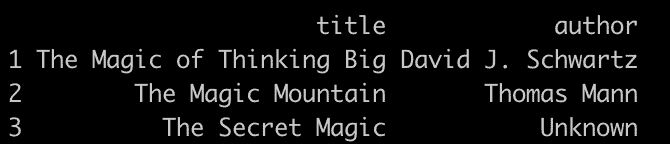# How to Use the str_detect() Function in R

The str_detect() function from the stringr in R is “used to detect the presence or absence of a specific pattern in a string.”

## Syntax

``str_detect(string, pattern, negate = FALSE)``

## Parameters

1. string: It is a character vector.
2. pattern: It is the pattern to look for.
3. negate: If TRUE, return non-matching elements.

## Example 1: Using str_detect() function with String

``````library(stringr)

fruits <- c("apple pie", "banana split", "cherry tart", "apple juice")

result <- str_detect(fruits, "apple")

print(result)
``````

Output

`````` TRUE FALSE FALSE TRUE
``````

## Example 2: Using Regular Expressions

You can detect if any strings in a vector start with the letter “a”.

``````library(stringr)

fruits <- c("apple", "banana", "avocado", "cherry")

result <- str_detect(fruits, "^a")

print(result)``````

Output

`` TRUE FALSE TRUE FALSE``

## Example 3: Case-Insensitive Detection

Detect the word “apple”, irrespective of its case.

``````library(stringr)

fruits <- c("Apple pie", "banana split", "APPLE juice", "cherry tart")

result <- str_detect(fruits, regex("apple", ignore_case = TRUE))

print(result)
``````

Output

`````` TRUE FALSE TRUE FALSE
``````

## Example 4: Using str_detect() with Vector

``````library(stringr)

# Create a vector of sentences
sentences <- c(
"The rain in Spain falls mainly on the plain.",
"It was a sunny day.",
"Raindrops keep falling on my head.",
"I don't have an umbrella."
)

# Use str_detect() to find sentences containing the word "rain"
contains_rain <- str_detect(sentences, "rain")

# Print the result
print(contains_rain)``````

Output

`` TRUE FALSE FALSE FALSE``

## Example 5: Using a str_detect() function with Data Frame

``````# Load the necessary libraries
library(stringr)
library(dplyr) # For data manipulation

# Create a data frame of books
books <- data.frame(
title = c(
"The Magic of Thinking Big",
"How to Win Friends and Influence People",
"The Magic Mountain",
"The Art of War",
"The Secret Magic"
),
author = c(
"David J. Schwartz",
"Dale Carnegie",
"Thomas Mann",
"Sun Tzu",
"Unknown"
)
)

# Use str_detect() to filter rows with
# titles containing the word "magic" (case-insensitive)
magic_books <- books %>%
filter(str_detect(title, regex("magic", ignore_case = TRUE)))

# Print the result
print(magic_books)``````

OutputThat’s it!

## Related posts

str_c() function in R

str_like() function in R

str() function in R

str_like() function in R

StrExtract() function in R